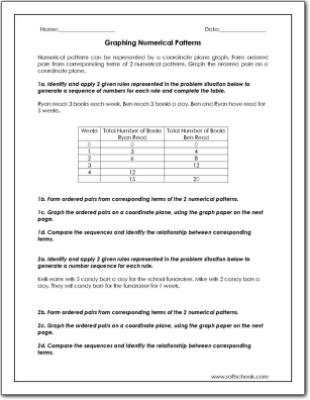# Graphing Numerical Patterns

Numerical patterns can be represented by a coordinate plane graph. Form ordered pairs from corresponding terms of 2 numerical patterns. Graph the ordered pairs on a coordinate plane.
Graphing Numerical Patterns